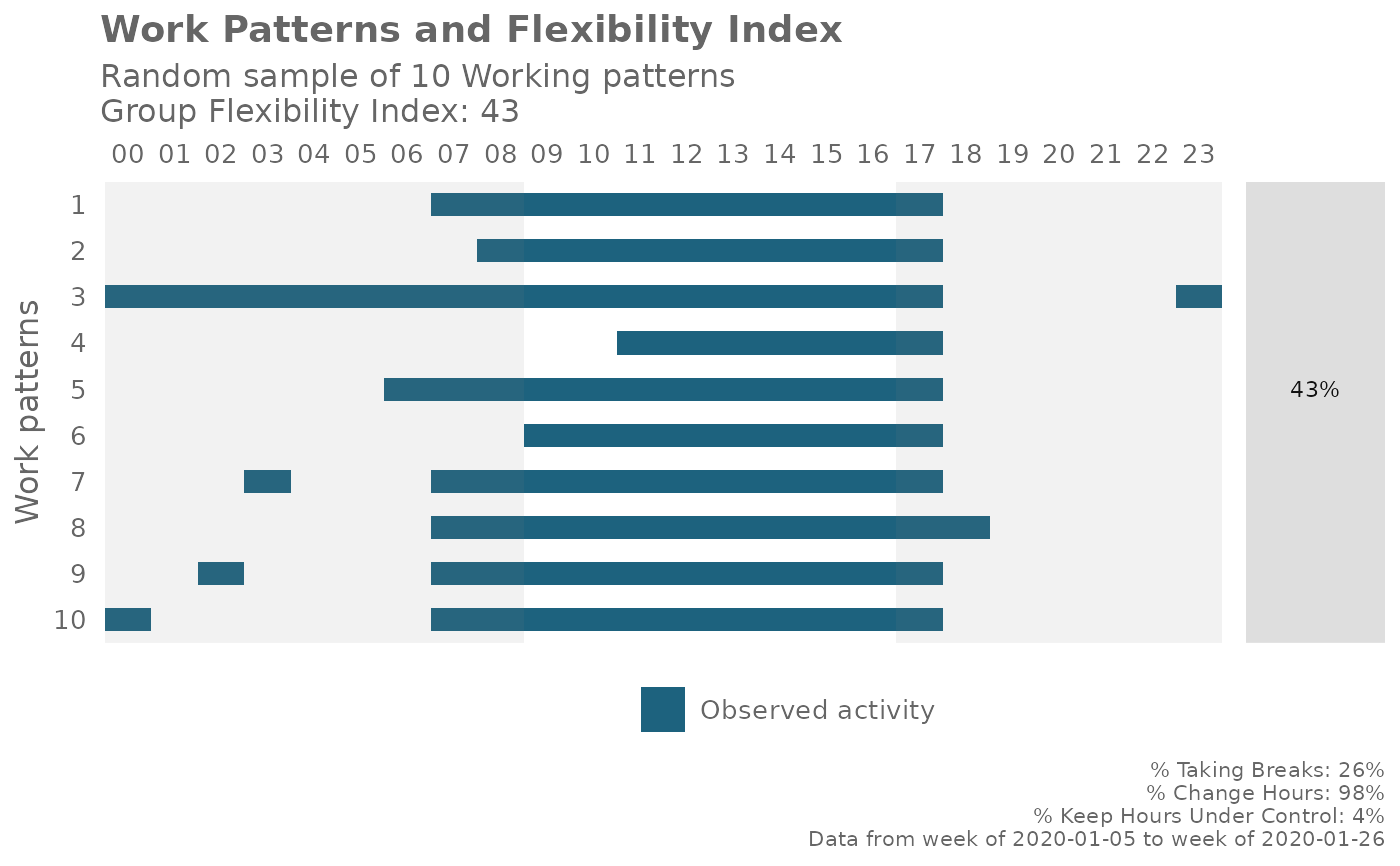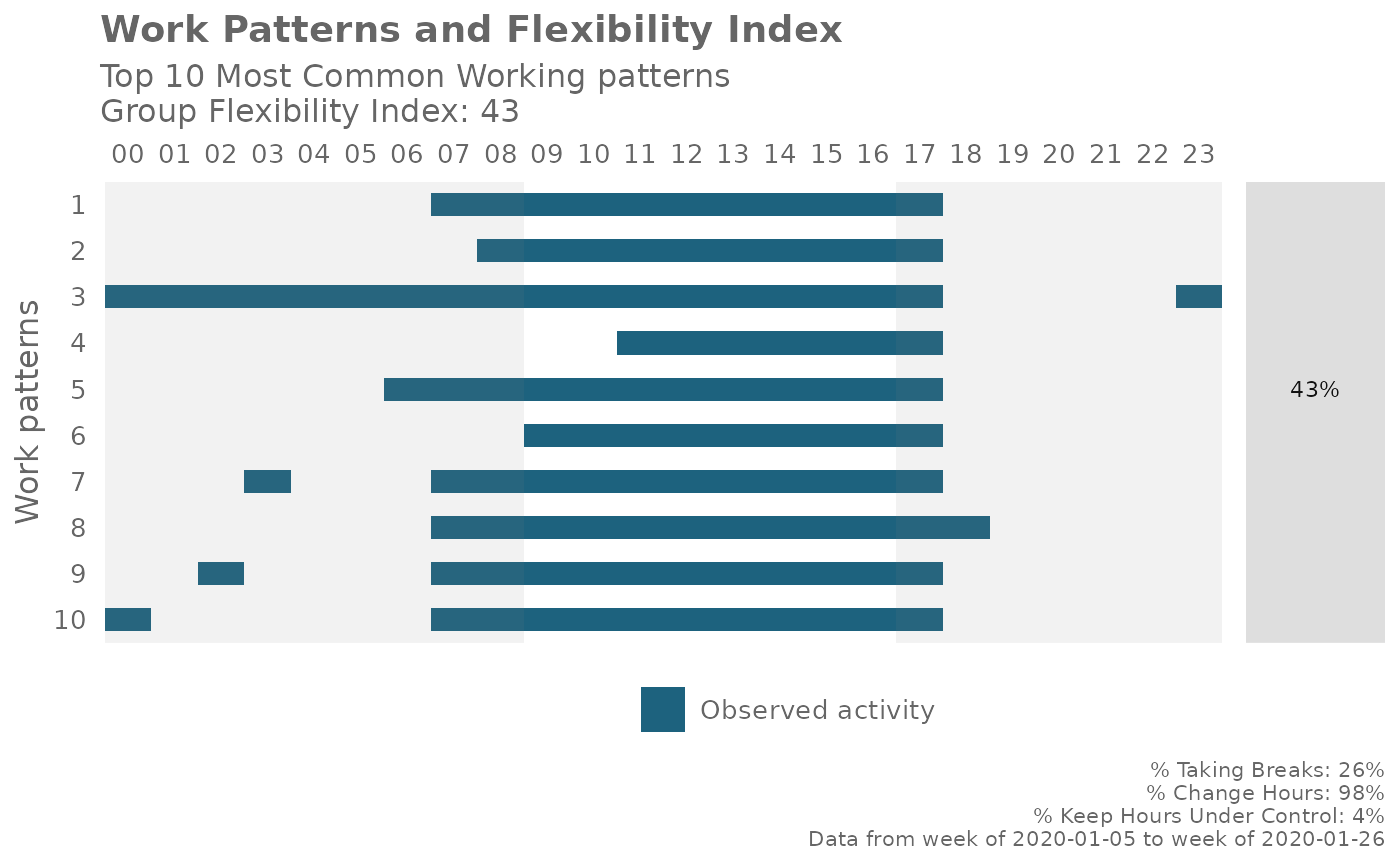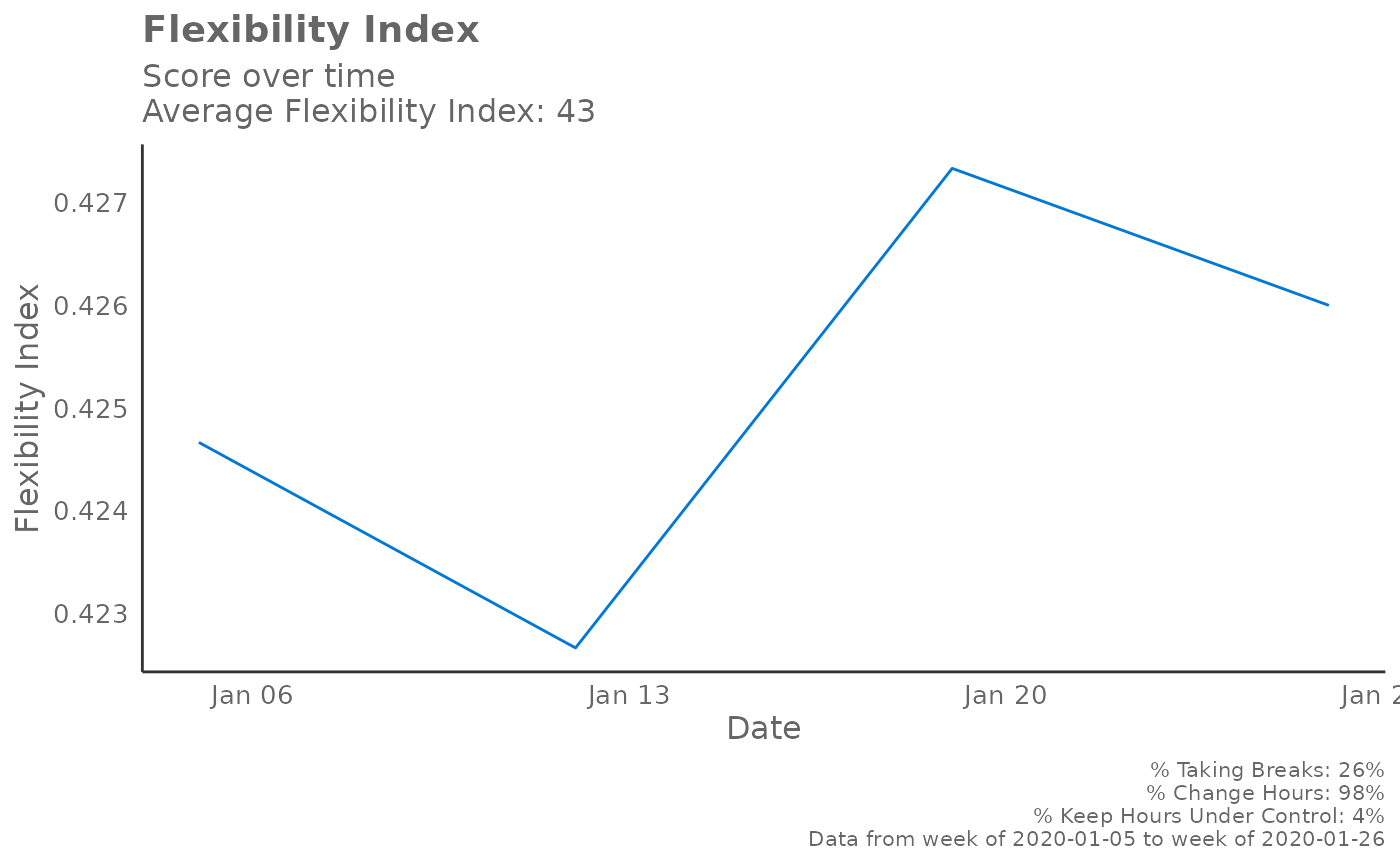This is a helper function for plotting visualizations for the Flexibility Index using the data output from flex_index(). This is used within flex_index() itself as an internal function.

plot_flex_index(
data,
sig_label = "Signals_sent_",
method = "sample",
start_hour = 9,
end_hour = 17,
mode = "binary"
)

## Arguments

data

Data frame. Direct data output from flex_index().

sig_label

Character string for identifying signal labels.

method

Character string for determining which plot to return. Options include "sample", "common", and "time". "sample" plots a sample of ten working patterns; "common" plots the ten most common working patterns; "time" plots the Flexibility Index for the group over time.

start_hour

See flex_index().

end_hour

See flex_index().

mode

See flex_index().

## Value

ggplot object. See method.

Other Working Patterns: flex_index(), identify_shifts_wp(), identify_shifts(), workpatterns_area(), workpatterns_classify_bw(), workpatterns_classify_pav(), workpatterns_classify(), workpatterns_hclust(), workpatterns_rank(), workpatterns_report()

## Examples

# Pre-calculate Flexibility Index
fi_output <- flex_index(em_data, return = "data")

# Examples of how to test the plotting options individually
# Sample of 10 work patterns
plot_flex_index(fi_output, method = "sample")# 10 most common work patterns
plot_flex_index(fi_output, method = "common")# Plot Flexibility Index over time
plot_flex_index(fi_output, method = "time")